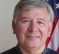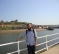## Predictive Analytics from Sensor DataStarted by 6 years ago12 replieslatest reply 6 years ago143 views

Hi All,

I have a time series data of time vs temperature and I am looking into temperature prediction.

But my question how can I model this problem? i.e. prediction using just one variable and what kind of techniques should be used?

Thanks!

Sia

[ - ]There are a lot of answers.  First, how much history do you have (or want)? How fast a response do you need?  If you have a lot of data and lot of time, the whole "deep learning" method can deal with a lot of exceptions to rules.  If you have a lot of data and not much time to respond, a Kalman filter might work.  If you don't have a lot of data and want a quick response, a PID controller can work fairly well.

Like everything, "it depends" is always the right answer :-)  If you want to start simple, look at the PID control method, and control theory in general.  As you get more complicated, the other things will appear as useful tools.

Dr. mike

[ - ]Thanks Mike!

I have fairly large data set of time vs temperature, approximately 75K samples taken over 4 days.

I would like to use R and as it is with such things, I do not have enough time. Let's say I have 7 days and if possible I would like to use either R or Python.

[ - ]You are getting 1 sample every 5 seconds so you have lots of time to do fancy things.  A polynomial curve fit of order 4 or 5 will be easy in R and give you about 1 days extrapolation.  If you go to higher order you should reduce the extrapolation distance (for example, you have more than enough data to give a 10th order polynomial, but only go 2 hours out because it will have some curvature that won't make sense beyond that).

https://en.wikipedia.org/wiki/Linear_least_squares...

Dr. mike

[ - ]Thank you Mike. But do I need to scale/convert the timestamps in order to do the LS fitting? For example, the data set is as follows:

pressure    datetime

4.848374    2016-04-12 10:04:00

4.683901    2016-04-12 10:04:32

5.237860    2016-04-12 10:13:20

[ - ]No, polynomial curve fitting can be done using arbitrary time steps.  I would suggest mapping the time into a single value.  For example, UNIX uses time from January 1 1970 as "the first epoch".  Using double precision floats you can keep track of seconds from some "zero time".  That makes all your time values monotonically increasing for as long as you need to run your program.  If you keep track of microseconds, you still have 100 years before you roll over, so it does not matter much when you pick zero time.  The Wolfram link shows how it works, as you go to higher order, inverting the matrix becomes more interesting.

Dr. mike

[ - ]Thank you Mike, I will try and implement this and report back!

[ - ]Consider these things:

1) Do you have a model of what's being measured?  For example, if it's a tank of 500,000 gallons of water then there is at least some thermal capacity, transfer of heat between the tank and the atmosphere and transfer of heat between the tank and outer space.  I only mention this because prediction is affected by physics.  And, of course, you'd want to know what the forcing functions might be.  So, you might predict the water temperature from the surrounding air temperature and be satisfied.  Or, you might predict the air temperature or use meteorological predictions as a forcing function (input) to your model.  Generally you might do better with a model.

2) But let's say there is no model.  Then all you have are a bunch of numbers that are aligned in time.  As a general statement:

a) The first thing to do is to remove any periodic components (such as diurnal temperature variations).

b) The second thing to do is to remove any trend component, i.e. straight line.

c) What we hope remains is a random sequence.  So at least accept this in principle.

Now, the best you can do with the random sequence is to predict that the next measurement will be the same as the last measurement.  Any other prediction method will generate higher errors I believe.  You can try it both ways and see for yourself.

Then you can add back in the periodic components and maybe the trend (but the latter is somewhat questionable and has to do with time frames.  i.e. a long time frame trend is likely better if you're going to do this than a series of short-term trends used one after another).

Once this is done you have the expected value of the next temperature or series of temperatures.  It's the best estimate.

Then, on top of this you may wish to know the expected distrubution of the outcomes.

Compute the variance of the random sequence and also match the random sequence to a distribution.  Gaussian and Weibull / Rayleigh are the two most common.  Use Gaussian if the distribution of outcomes is independent of the zero point.  You might use Weibull if the outcomes are always positive (or always negative); i.e. never cross the zero point.

Then you can use random number generators to determine *CASES* of possible outcomes that are centered on the expected values.  Run a suitable number of such cases and you'll have a distribution of possible outcomes along with an estimate of their probabilities.

Curve fitting to get future data point predictions doesn't have a place in this it seems to me.  Without a model, it can only generate higher errors.

[ - ]Considering the sampling rate and Using polynomial nature of the signal, use different predictive tools to plot future trend (extrapolate) from the available data.

[ - ]Hi,

You can use linear prediction (google it:). I think it is the most basic. However, the prediction method depends on the underlying model of the data. As the model gets complicated, the algorithm gets too. So as a start assume that the model is first order auto-regressive (AR), so you have only one parameter to estimate here (a(1)). Find it then try to predict the data.

May I ask, out of curiosity, why do you want to predict the temprature?

[ - ]You cannot have a prediction unless you have some sort of a model to work from.  To use some ad-hoc technique is to implicitly assume a model.

If you assume that the temperature will match previous values, then you're assuming a single integrator driven by some white random process.  If you assume that the temperature will match the previous trend, then you're assuming a double integrator driven by some white random process.  Assuming that it matches some daily periodicity assumes some more complicated model yet.

Whatever you do, the accuracy of your prediction will go down the farther out you try to predict.  If you're trying to predict outdoor temperatures, then any blind model will, eventually, fail spectacularly, because weather is weather.

[ - ]You can implement a sample-by-sample simple predictor using an alpha filter, i.e.

x=x+alpha*(z-x)

where, 0<alpha<1 is the fixed gain, and 'z' is the input sample. You need to find a good value for alpha, and perhaps make alpha dynamic. You may of course expand this model by including rate of change (the so called alpha-beta filter).

I think that Tim has a valid point that you really need to understand what your process is, and design an appropiate model in order to get any good performance. We use this model for several tracking applications rather than a more computationally expensive Kalman filter, but first run a few simulations with a KF in order to get an idea about the value of alpha.

[ - ]